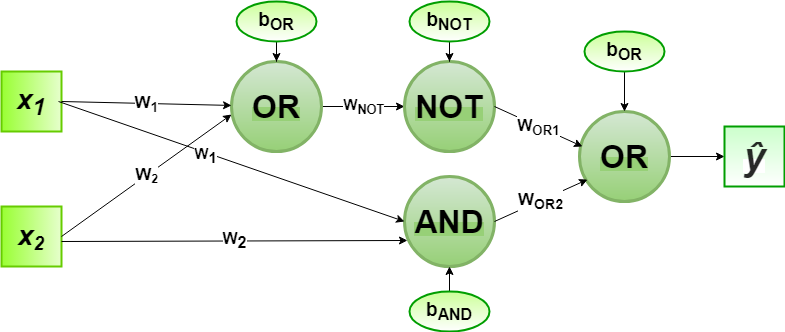GeeksforGeeks App
Open AppBrowser
Continue

# Implementation of Perceptron Algorithm for XNOR Logic Gate with 2-bit Binary Input

In the field of Machine Learning, the Perceptron is a Supervised Learning Algorithm for binary classifiers. The Perceptron Model implements the following function:For a particular choice of the weight vectorand bias parameter, the model predicts outputfor the corresponding input vector. XNOR logical function truth table for 2-bit binary variables, i.e, the input vectorand the corresponding outputWe can observe that,Designing the Perceptron Network:

1. Step1: Now for the corresponding weight vectorof the input vectorto the OR and AND node, the associated Perceptron Function can be defined as:[Tex]$\boldsymbol{\hat{y}_{2}} = \Theta\left(w_{1} x_{1}+w_{2} x_{2}+b_{AND}\right)$ [/Tex]

2. Step2: The outputfrom the OR node will be inputted to the NOT node with weightand the associated Perceptron Function can be defined as:3. Step3: The outputfrom the AND node and the outputfrom NOT node as mentioned in Step2 will be inputted to the OR node with weight. Then the corresponding outputis the final output of the XNOR logic function. The associated Perceptron Function can be defined as:For the implementation, the weight parameters are considered to beand the bias parameters are. Python Implementation:

## Python3

 # importing Python libraryimport numpy as np # define Unit Step Functiondef unitStep(v):    if v >= 0:        return 1    else:        return 0 # design Perceptron Modeldef perceptronModel(x, w, b):    v = np.dot(w, x) + b    y = unitStep(v)    return y # NOT Logic Function# wNOT = -1, bNOT = 0.5def NOT_logicFunction(x):    wNOT = -1    bNOT = 0.5    return perceptronModel(x, wNOT, bNOT) # AND Logic Function# w1 = 1, w2 = 1, bAND = -1.5def AND_logicFunction(x):    w = np.array([1, 1])    bAND = -1.5    return perceptronModel(x, w, bAND) # OR Logic Function# here w1 = wOR1 = 1,# w2 = wOR2 = 1, bOR = -0.5def OR_logicFunction(x):    w = np.array([1, 1])    bOR = -0.5    return perceptronModel(x, w, bOR) # XNOR Logic Function# with AND, OR and NOT # function calls in sequencedef XNOR_logicFunction(x):    y1 = OR_logicFunction(x)    y2 = AND_logicFunction(x)    y3 = NOT_logicFunction(y1)    final_x = np.array([y2, y3])    finalOutput = OR_logicFunction(final_x)    return finalOutput # testing the Perceptron Modeltest1 = np.array([0, 1])test2 = np.array([1, 1])test3 = np.array([0, 0])test4 = np.array([1, 0]) print("XNOR({}, {}) = {}".format(0, 1, XNOR_logicFunction(test1)))print("XNOR({}, {}) = {}".format(1, 1, XNOR_logicFunction(test2)))print("XNOR({}, {}) = {}".format(0, 0, XNOR_logicFunction(test3)))print("XNOR({}, {}) = {}".format(1, 0, XNOR_logicFunction(test4)))

Output:

XNOR(0, 1) = 0
XNOR(1, 1) = 1
XNOR(0, 0) = 1
XNOR(1, 0) = 0

Here, the model predicted output () for each of the test inputs are exactly matched with the XNOR logic gate conventional output () according to the truth table. Hence, it is verified that the perceptron algorithm for XNOR logic gate is correctly implemented.

My Personal Notes arrow_drop_up